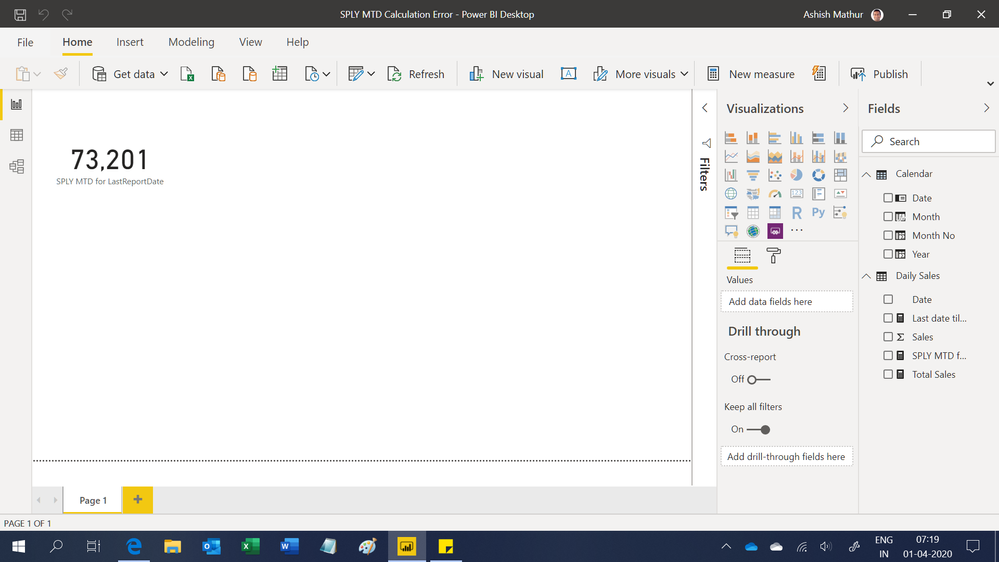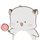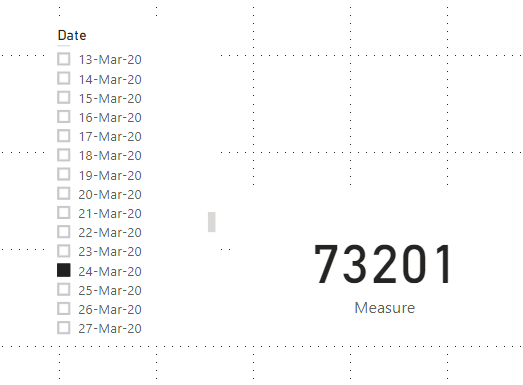cancel
Showing results for
Did you mean:Helper I

## MTD - Same Period Last Year with Missing Dates

Hi All,

I need to create a measure to calculate the MTD Sales for the same period last year based on the latest daily sales report saved in the sales table.

I have 2 tables, one a daily sales table and a calendar table for this. Please find HERE a sample PowerBI file if needed

I have written the measure:

SPLY MTD for LastReportDate = CALCULATE([Total Sales],DATESMTD(DATEADD(LASTDATE('Daily Sales'[Date]),-1,YEAR)),ALLEXCEPT('Daily Sales','Daily Sales'[Date]))

This works beautifully until I have a date (e.g. 24 March 2020) which will fall on a non-working day in the previous year.

The correct outcome from the measure for SPLY MTD as at 24 Mar 2020 should be 73,201

1 ACCEPTED SOLUTIONSuper User

Hi,

Hope this helps.Regards,
Ashish Mathur
http://www.ashishmathur.com
3 REPLIES 3Super User

For time intelligence-related stuff you should use a date calendar, you date from the calendar

Example

``````MTD Sales = CALCULATE(SUM(Sales[Sales Amount]),DATESMTD('Date'[Date]))
last MTD Sales = CALCULATE(SUM(Sales[Sales Amount]),DATESMTD(dateadd('Date'[Date],-1,MONTH)))
last MTD (complete) Sales =  CALCULATE(SUM(Sales[Sales Amount]),DATESMTD(ENDOFMONTH(dateadd('Date'[Date],-1,MONTH))))
last year MTD Sales = CALCULATE(SUM(Sales[Sales Amount]),DATESMTD(dateadd('Date'[Date],-12,MONTH)))
last year MTD (complete) Sales =  CALCULATE(SUM(Sales[Sales Amount]),DATESMTD(ENDOFMONTH(dateadd('Date'[Date],-12,MONTH))))

last QTR same Month (complete) Sales =  CALCULATE(SUM(Sales[Sales Amount]),DATESMTD(ENDOFMONTH(dateadd('Date'[Date],-1,Qtr))))

MTD (Year End) Sales =  CALCULATE(SUM(Sales[Sales Amount]),DATESMTD(ENDOFYEAR('Date'[Date])))
MTD (Last Year End) Sales =  CALCULATE(SUM(Sales[Sales Amount]),DATESMTD(ENDOFYEAR(dateadd('Date'[Date],-12,MONTH),"8/31")))

``````

To get the best of the time intelligence function. Make sure you have a date calendar and it has been marked as the date in model view. Also, join it with the date column of your fact/s. Refer :
https://www.archerpoint.com/blog/Posts/creating-date-table-power-bi
https://www.sqlbi.com/articles/creating-a-simple-date-table-in-dax/Community Support

Hi,

1)Delete the relationship between them:2)Create a calculated column in 'Daily Sales' table:

``Year&Month = FORMAT('Daily Sales'[Date],"YYYY-MMM")``

3)Try this measure:

``````Measure =
VAR a =
SELECTEDVALUE ( 'Calendar'[Date] )
RETURN
SUMX (
GROUPBY ( 'Daily Sales', 'Daily Sales'[Date], 'Daily Sales'[Year&Month] ),
CALCULATE (
IF (
MAX ( 'Daily Sales'[Date] )
>= DATE ( YEAR ( a ) - 1, MONTH ( a ), 1 )
&& MAX ( 'Daily Sales'[Date] )
<= DATE ( YEAR ( a ) - 1, MONTH ( a ), DAY ( a ) ),
CALCULATE (
SUM ( 'Daily Sales'[Sales] ),
FILTER (
'Daily Sales',
[Date] <= MAX ( 'Daily Sales'[Date] )
&& [Year&Month] IN FILTERS ( 'Daily Sales'[Year&Month] )
)
),
0
)
)
)``````

4)Choose this measure as a card visual, 'Calendar'[Date] as a slicer.

When select March 24 in slicer, the result shows:Here is the test pbix file:

Hope this helps.

Best Regards,

Giotto ZhiSuper User

Hi,

Hope this helps.Regards,
Ashish Mathur
http://www.ashishmathur.com